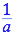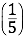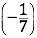# Counting by Piles

## OBJECTIVE: To learn about the axioms of multiplication

In previous lessons, you learned about AXIOMS OF EQUALITY and AXIOMS OF ADDITION. Keeping Your Balance: https://www.geogebra.org/m/v5jyuhxm Mixing Things Together: https://www.geogebra.org/m/fmktaxpa In this lesson, you're going to learn about the AXIOMS OF MULTIPLICATION. The following axioms define the rules for multiplying real numbers. 1. CLOSURE AXIOM FOR MULTIPLICATION — If a and b are real numbers, then a•b is equal to a unique real number, i.e., if two real numbers are multiplied together, the product is a real number. 2. IDENTITY AXIOM FOR MULTIPLICATION — For any real number a, a•1 = a or 1a = a. One ( 1 ) is the identity element for multiplication. 3. MULTIPLICATIVE INVERSE FOR MULTIPLICATION — Every real number a has a multiplicative inversesuch that a•= 1 or•a = 1. 4. COMMUTATIVE AXIOM FOR MULTIPLICATION — If a and b are real numbers, then a•b = b•a, i.e., the order in which two or more numbers are multiplied together does not affect the product. 5. ASSOCIATIVE AXIOM FOR MULTIPLICATION — If a, b, and c are real numbers, then (a•b)•c = a•(b•c); i.e., the grouping of three or more numbers for multiplication does not affect the product. 6. MULTIPLICATION BY ZERO — If a is any real number, then a0 = 0 or 0•a = 0. 7. DISTRIBUTIVE AXIOM OF MULTIPLICATION — If a, b, and c are real numbers, then a(b + c) = a•b + a•c or a(b - c) = a•b - a•c; i.e., a is OVER ADDITION AND SUBTRACTION distributed across the sum or difference of b and c. Algebraic Examples: 1. CLOSURE AXIOM FOR MULTIPLICATION — 57 = 35 Since 5 and 7 are real numbers, 35 is also a real number. 2. IDENTITY AXIOM FOR MULTIPLICATION — 101 = 10 & 1(-12) = -12 3. MULTIPLICATIVE INVERSE FOR MULTIPLICATION — 5= 1 & (-7)= 1 4. COMMUTATIVE AXIOM FOR MULTIPLICATION — 23 = 6 32 = 6 5. ASSOCIATIVE AXIOM FOR MULTIPLICATION — (23)4 = 64 = 24 2(34) = 212 = 24 6. MULTIPLICATION BY ZERO — 80 = 0 & 08 = 0 7. DISTRIBUTIVE AXIOM OF MULTIPLICATION OVER ADDITION AND SUBTRACTION — 2(x + y) = 2x + 2y & 2(x - y) = 2x - 2y
Below is a set of problems involving axioms of multiplication.

## ANSWER BOX:

Check your answers below.

## In this lesson, you learned about the axioms of multiplication.

In future lessons, you'll learn about order of operations for real numbers. Did you ENJOY today's lesson?